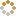﻿ Ningbo University of Technology Online Judge :: NBUT 2015 Timed NOJ Training #010 :: Find Sum
• ### [A] Find Sum

• 时间限制: 1000 ms　内存限制: 65535 K
• 问题描述
• This problem is really boring.

You are given a number sequence S which contain n integers and m queries, each time i will give your two integers id1 and id2, you need to tell me whether i can get S[id1] + S[id2] from the n integers.

• 输入
• Input starts with an integer T(T <= 5) denoting the number of test cases.
For each test case, n(1 <= n <= 100000, 1 <= m <= 10000) are given, and the second line contains n integers, each of them is larger then 0 and no larger than 10^11.
Next m lines, each line contains two integers id1 and id2(1 <= id1, id2 <= n).
• 输出
• For each case, first print the case number.
For each query, print “Yes” if i can get S[id1] + S[id2] from S, otherwise print “No”(without the quote).
• 样例输入
• ```1
5 3
3 4 2 1 3
1 2
3 4
4 5
```
• 样例输出
• ```Case 1:
No
Yes
Yes
```
• 提示
• `无`
• 来源
• `Alex@NBUT`
• 操作
﻿